2022-2-17 16:47
22482

# 前置小知识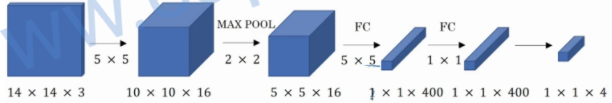14x14表示有14x14个像素，3表示RGB三种颜色，从图片的变化来看，每张图片的像素变少了，但是层数增加了，最后从400变成了4是因为最后加了一层全连接层，至于到底为什么这么做能使图像训练效率变高，至今也没有一个准确的定义，大致的思路就是在图像中的一些大数字代表了某种含义（这里用的池化层是最大池化层），将这些大数字保留下来，过滤掉小数字，就可以过滤掉一些图片中不重要的信息，从而减少训练量（卷积+池化可以有效的减少算力成本）；至于残差网络，是用来防止梯度爆炸和梯度消失问题的，在训练的时候会因为层数的增加导致在梯度下降的时候产生梯度消失（与x轴水平）或梯度爆炸（与y轴水平）的问题，为了解决这个问题，何凯明、张翔宇、任少卿和孙剑这四位大佬设计出了残差网络，残差网络说白了就是为激活值提供一条小路，将前面的激活值带到后面层中，这样就解决了梯度爆炸和梯度消失的问题（从训练结果来看也确实是有效的）；这些前置知识在本例中只需要掌握大概原理就行了，并不需要自己动手从头写一个，卷积、池化、全连接和残差网络这几项技术都是需要许多的数学与算法基础的，并非明白原理就能直接写出

# 我的代码

## yolo介绍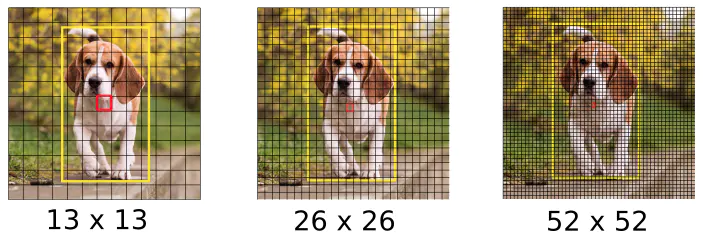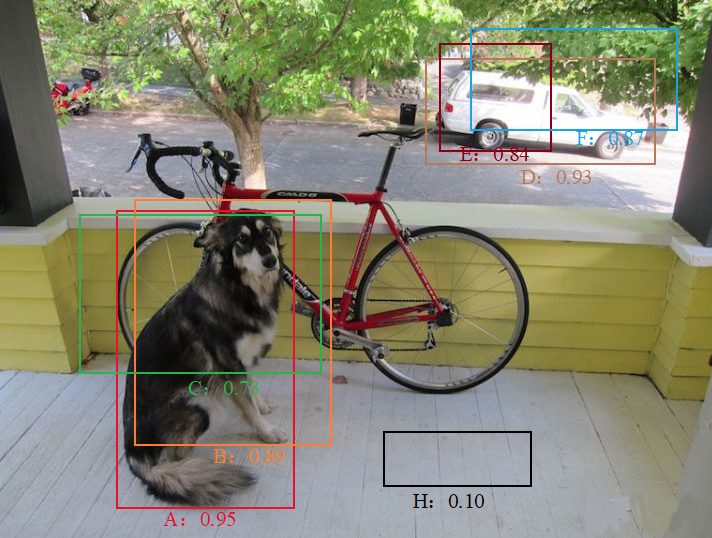## 代码部分

```import numpy as np
import tensorflow as tf
import cv2
from IPython.display import Image,display
%matplotlib inline```
```###############################################################################################
# 过滤概率低的边框
# 参数：
# box_confidence：装载着每个边框的pc
# boxes：装载着每个边框的坐标
# box_class_probs：装载着每个边框的80个种类的概率
# threshold：阈值，概率低过这个值的边框会被过滤掉
#
# 返回值：
# scores：装载保留下的那些边框的概率
# boxes：装载保留下的那些边框的坐标
# classes：装载保留下的那些边框的种类的索引
###############################################################################################
def yolo_filter_boxes(box_confidence,boxes,box_class_probs,threshold=.6):
# 将pc和c相乘，得到具体某个种类是否存在的概率
box_scores=box_confidence*box_class_probs

# 获取概率最大的那个种类的索引
box_classes=tf.argmax(box_scores,axis=-1)

# 获取概率最大的那个种类的概率值
box_class_scores=tf.reduce_max(box_scores,axis=-1)

# 用上面的过滤器过滤掉那些概率小的边框
# 过滤完成后，scores和boxes，classes里面就只装载了概率大的边框的概率值和坐标以及种类索引了
return scores,boxes,classes

# 模块测试
box_confidence=tf.random.normal([13,13,3,1],mean=1,stddev=4,seed=1)
boxes=tf.random.normal([13,13,3,4],mean=1,stddev=4,seed=1)
box_class_probs=tf.random.normal([13,13,3,80],mean=1,stddev=4,seed=1)
scores,boxes,classes=yolo_filter_boxes(box_confidence,boxes,box_class_probs,0.5)
print("scores=",scores)
print("boxes=",boxes)
print("classes=",classes)
print("scores.shape=",scores.shape)
print("boxes.shape=",boxes.shape)
print("classes.shape=",classes.shape)```

scores= tf.Tensor(12.552861, shape=(), dtype=float32)

boxes= tf.Tensor([ 3.8289614   0.14167517 -0.03989506 -3.3593693 ], shape=(4,), dtype=float32)

classes= tf.Tensor(46, shape=(), dtype=int64)

scores.shape= (500,)

boxes.shape= (500, 4)

classes.shape= (500,)

```###############################################################################################
# 用非最大值抑制技术过滤掉重叠的边框
# 参数：
# scores：前面yolo_filter_boxes函数保留下的那些边框的概率值
# boxes：前面yolo_filter_boxes函数保留下的那些边框的坐标
# classes：前面yolo_filter_boxes函数保留下的那些边框的种类的索引
# max_boxes：最多想要保留多少个边框
# iou_threshold：交并比阈值，交并比大于这个阈值的边框才会被进行非最大值抑制处理
#
# 返回值：
# scores：NMS保留下的那些边框的概率
# boxes：NMS保留下的那些边框的坐标
# classes：NMS保留下的那些边框的种类的索引
###############################################################################################
def yolo_non_max_suppression(scores,boxes,classes,max_boxes=20,iou_threshold=0.5):
# NMS函数，此函数会返回NMS后保留下来的边框的索引
nms_indices=tf.image.non_max_suppression(boxes,scores,max_boxes,iou_threshold=iou_threshold)

# 通过上面的索引来分别获取被保留的边框的相关概率值、坐标以及种类的索引
scores=tf.gather(scores,nms_indices)
boxes=tf.gather(boxes,nms_indices)
classes=tf.gather(classes,nms_indices)
return scores,boxes,classes

# 模块测试
scores=tf.random.normal([54,],mean=1,stddev=4,seed=1)
boxes=tf.random.normal([54,4],mean=1,stddev=4,seed=1)
classes=tf.random.normal([54,],mean=1,stddev=4,seed=1)
scores,boxes,classes=yolo_non_max_suppression(scores,boxes,classes)
print("scores=",scores)
print("boxes=",boxes)
print("classes=",classes)
print("scores.shape=",scores.shape)
print("boxes.shape=",boxes.shape)
print("classes.shape=",classes.shape)```

scores= tf.Tensor(8.208248, shape=(), dtype=float32)

boxes= tf.Tensor([ 5.8494906  -0.32543743  0.8039762  -3.6349177 ], shape=(4,), dtype=float32)

classes= tf.Tensor(-5.3616023, shape=(), dtype=float32)

scores.shape= (20,)

boxes.shape= (20, 4)

classes.shape= (20,)

```###############################################################################################
# 最终的过滤函数
# 参数：
# yolo_outputs：YOLO模型的输出结果
# max_boxes：你希望最多识别出多少个边框
# score_threshold：概率值阈值
# iou_threshold：交并比阈值
#
# 返回值：
# scores：最终保留下的那些边框的概率
# boxes：最终保留下的那些边框的坐标
# classes：最终保留下的那些边框的种类的索引
###############################################################################################
def yolo_eval(outputs,max_boxes=20,score_threshold=0.5,iou_threshold=0.5):
# 建立3个空list
s,b,c=[],[],[]

# 后面调用的Yolov3使用了3个规格的网格(13*13，26*26，52*52)进行预测，所以有三组output
for output in outputs:
# 将YOLO输出结果分成3份，分别表示概率值、坐标、种类索引
box_confidence,boxes,box_class_probs=output
# 使用之前实现的yolo_filter_boxes函数过滤掉概率值低于阈值的边框
scores,boxes,classes=yolo_filter_boxes(box_confidence,boxes,box_class_probs,threshold=score_threshold)
s.append(scores)
b.append(boxes)
c.append(classes)

# 将3组output的结果整合到一起
scores=tf.concat(s,axis=0)
boxes=tf.concat(b,axis=0)
classes=tf.concat(c,axis=0)

# 使用yolo_non_max_suppression过滤掉重叠的边框
scores,boxes,classes=yolo_non_max_suppression(scores,boxes,classes,max_boxes=max_boxes,
iou_threshold=iou_threshold)
return scores,boxes,classes

yolo_output=(tf.random.normal([13,13,3,1],mean=1,stddev=4,seed=1),
tf.random.normal([13,13,3,4],mean=1,stddev=4,seed=1),
tf.random.normal([13,13,3,80],mean=1,stddev=4,seed=1))
yolo_output1=(tf.random.normal([26,26,3,1],mean=1,stddev=4,seed=2),
tf.random.normal([26,26,3,4],mean=1,stddev=4,seed=2),
tf.random.normal([26,26,3,80],mean=1,stddev=4,seed=2))
yolo_output2=(tf.random.normal([52,52,3,1],mean=1,stddev=4,seed=3),
tf.random.normal([52,52,3,4],mean=1,stddev=4,seed=3),
tf.random.normal([52,52,3,80],mean=1,stddev=4,seed=3))

# 模块测试
yolo_outputs=(yolo_output,yolo_output1,yolo_output2)
scores,boxes,classes=yolo_eval(yolo_outputs)
print("scores=",scores)
print("boxes=",boxes)
print("classes=",classes)
print("scores.shape=",scores.shape)
print("boxes.shape=",boxes.shape)
print("classes.shape=",classes.shape)```

scores= tf.Tensor(183.36862, shape=(), dtype=float32)

boxes= tf.Tensor([-0.9321569  1.2601769 -0.5666194 -1.3579395], shape=(4,), dtype=float32)

classes= tf.Tensor(23, shape=(), dtype=int64)

scores.shape= (20,)

boxes.shape= (20, 4)

classes.shape= (20,)

```# 定义种类已经anchor box和像素

# 加载已经训练好的YOLO模型
yolo_model.summary()```

__________________________________________________________________________________________________

Layer (type)                    Output Shape         Param #     Connected to

============================================================

input (InputLayer)              [(None, 416, 416, 3) 0

__________________________________________________________________________________________________

yolo_darknet (Functional)       [(None, None, None,  40620640    input

__________________________________________________________________________________________________

yolo_conv_0 (Functional)        (None, 13, 13, 512)  11024384    yolo_darknet

__________________________________________________________________________________________________

yolo_conv_1 (Functional)        (None, 26, 26, 256)  2957312     yolo_conv_0

yolo_darknet

__________________________________________________________________________________________________

yolo_conv_2 (Functional)        (None, 52, 52, 128)  741376      yolo_conv_1

yolo_darknet

__________________________________________________________________________________________________

yolo_output_0 (Functional)      (None, None, None, 3 4984063     yolo_conv_0

__________________________________________________________________________________________________

yolo_output_1 (Functional)      (None, None, None, 3 1312511     yolo_conv_1

__________________________________________________________________________________________________

yolo_output_2 (Functional)      (None, None, None, 3 361471      yolo_conv_2

============================================================

Total params: 62,001,757

Trainable params: 61,949,149

Non-trainable params: 52,608

__________________________________________________________________________________________________

```# 探测图片
img_raw,img=preprocess_image("test.jpg",model_image_size=(416,416))
yolo_outputs=yolo_model(img)

# 将YOLO模型的输出结果转换成我们需要的格式

# 过滤边框
out_scores,out_boxes,out_classes=yolo_eval(outputs)```
```# 加载图片并进行测试
def img_show(image_file,out_scores,out_boxes,out_classes,class_names):
img=cv2.cvtColor(img_raw.numpy(),cv2.COLOR_RGB2BGR)
colors=generate_colors(class_names)

# print('在{}图片中找到{}个目标'.format(image_file),len(out_boxes))
print('Found {} boxes for {}'.format(len(out_boxes),image_file))
img=draw_outputs(img,out_scores,out_boxes,out_classes,colors,class_names)
display(Image(data=bytes(cv2.imencode('.jpg',img)),width=800))
file_name=[x for x in image_file.split('.')]
cv2.imwrite('./res/'+file_name+'_out.'+file_name,img)
return img

# 使用测试训练集进行检测
img=img_show('test.jpg',out_scores,out_boxes,out_classes,class_names)```

Found 12 boxes for test.jpg

car 1.00 (353, 295) (755, 645)

car 1.00 (761, 286) (923, 404)

car 1.00 (944, 322) (1288, 701)

car 0.99 (925, 294) (1054, 379)

car 0.99 (142, 304) (330, 449)

car 0.91 (709, 281) (778, 347)

car 0.88 (309, 303) (366, 355)

car 0.80 (385, 291) (437, 325)

car 0.75 (673, 279) (720, 321)

traffic light 0.70 (680, 194) (690, 210)

car 0.68 (965, 272) (1025, 290)

truck 0.60 (579, 247) (644, 298)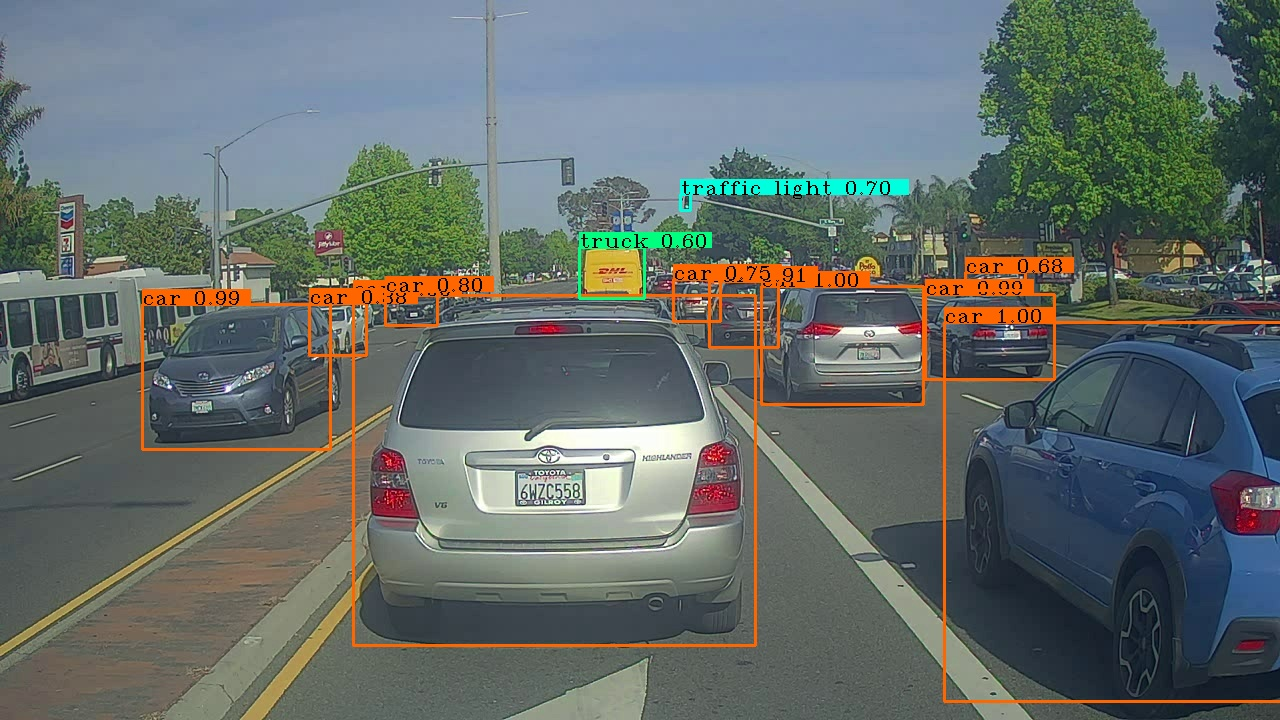```# 对目标图片进行预测
def predict(model,image_file,anchors,class_names):
img_raw,img=preprocess_image(image_file,model_image_size=(416,416))
yolo_outputs=yolo_model(img)
out_scores,out_boxes,out_classes=yolo_eval(outputs)
img=img_show(image_file,out_scores,out_boxes,out_classes,class_names)
return img

img=predict(yolo_model,'ID_6ae2b25af.jpg',anchors,class_names)```

Found 15 boxes for ID_6ae2b25af.jpg

car 0.99 (2062, 1859) (2516, 2231)

car 0.98 (1964, 1826) (2218, 2007)

car 0.98 (-11, 1759) (335, 1966)

car 0.94 (1859, 1780) (2074, 1925)

car 0.88 (664, 2372) (3213, 2711)

car 0.78 (1502, 1734) (1576, 1791)

car 0.72 (308, 1704) (428, 1787)

car 0.71 (707, 1712) (814, 1765)

car 0.71 (1758, 1748) (1842, 1823)

car 0.70 (568, 1718) (694, 1769)

car 0.64 (443, 1708) (568, 1770)

car 0.62 (2254, 1769) (2367, 1834)

car 0.60 (1802, 1757) (1895, 1832)

car 0.57 (2177, 1767) (2286, 1831)

car 0.51 (833, 1697) (939, 1758)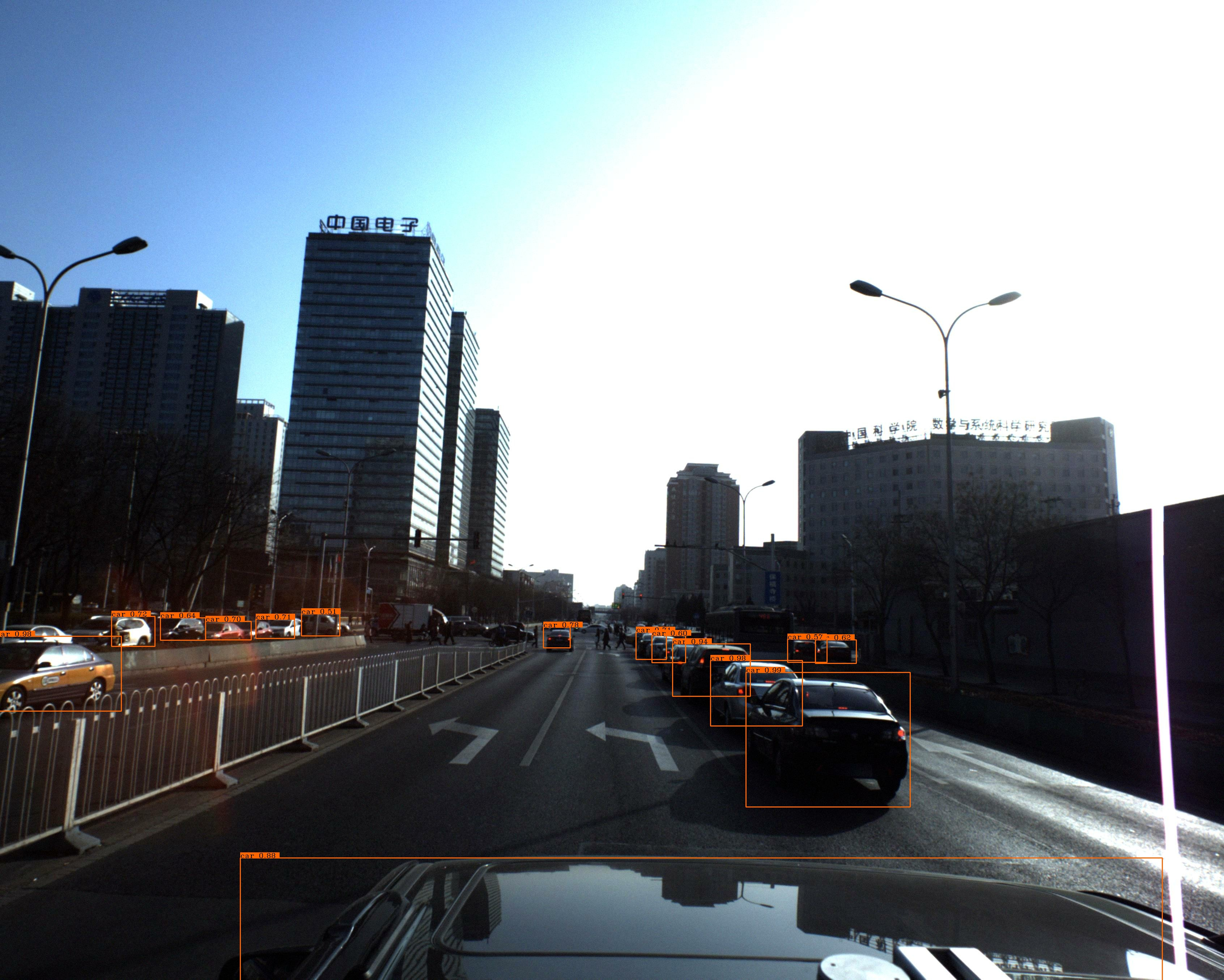```import os

Epath=os.walk('./images')
for path,dir,filelist in Epath:
for filename in filelist:
img_path = os.path.join(filename)
#print(img_path)
img=predict(yolo_model,img_path,anchors,class)```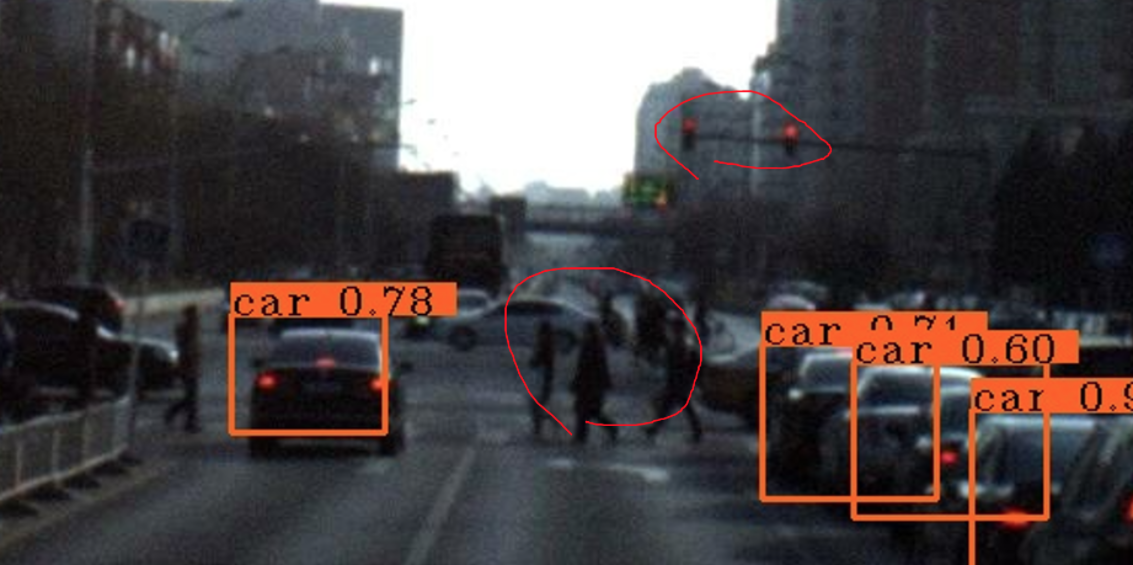# 结束语

https://zhuanlan.zhihu.com/p/76802514

https://www.jianshu.com/p/043966013dde

https://www.cnblogs.com/chenhuabin/p/13908615.html

4h入门PHP代码审计之反序列化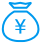打赏次数 1 雪花 + 50.00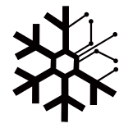Editor 50 2022/03/21 恭喜您获得“雪花”奖励，安全圈有你而精彩！pureGavin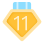2 2022-2-17 17:33
2
0obaby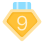20 2022-2-17 17:44
3
0

```# 模型效果测试
import os
with open('data/ImageSets/test.txt', "r") as f:
cmd = 'python detect_ads.py --source data/images/' +line.strip()+ '.jpg'
os.system(cmd)```pureGavin2 2022-2-17 17:48
4
0obaby 终于对yolo下手了，写个代码循环跑啊。哈哈。我是完全没考虑效率问题：#&nbsp;模型效果测试 import&nbsp;os with&nbsp;open(&#3 ...pureGavin2 2022-2-17 17:57
5
0obaby 终于对yolo下手了，写个代码循环跑啊。哈哈。我是完全没考虑效率问题：#&nbsp;模型效果测试 import&nbsp;os with&nbsp;open(&#3 ...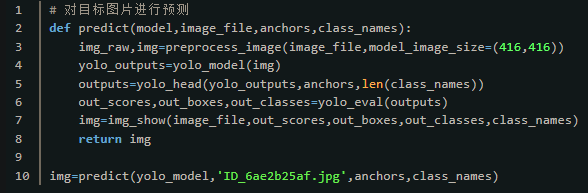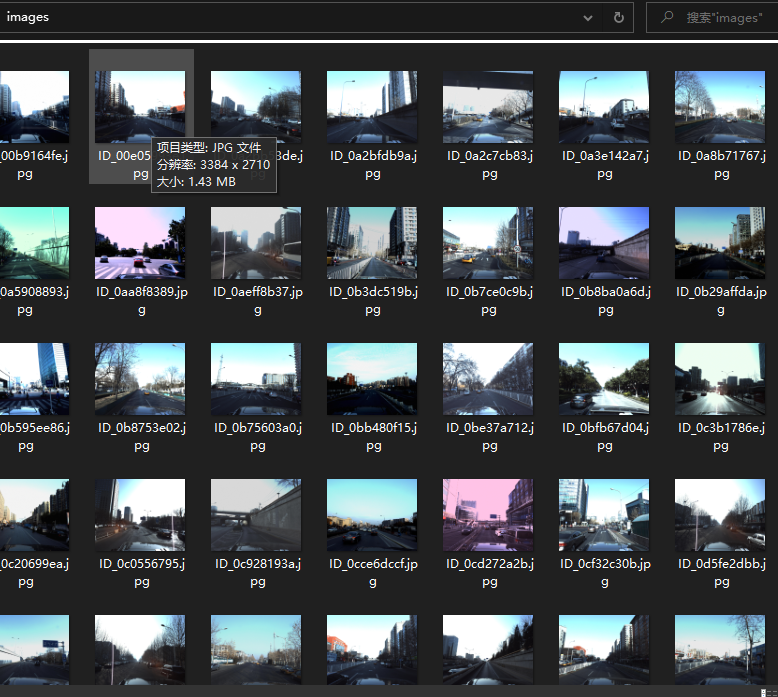obaby20 2022-2-17 18:20
6
0pureGavin 你这个好像不行，我是想在文章的最后这个部分加一个目录下图片遍历的代码目录中的图片大致是这样的
```Epath=os.walk('c://images')
for path,dir,filelist in Epath:
for filename in filelist:
img_path = os.path.join(path,filename)
img=predict(yolo_model,img_path,anchors,class_names)```pureGavin2 2022-2-18 09:52
7
0obaby pureGavin 你这个好像不行，我是想在文章的最后这个部分加一个目录下图片遍历的代码目录中的图片大致是这样的 Epath=os.walk(& ...KingSelyF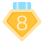1 2022-2-21 09:48
8
0pureGavin2 2022-2-21 10:16
9
0KingSelyF 佬，带带弟弟Nerium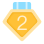2022-3-8 19:15
10
0pureGavin2 2022-3-9 09:32
11
0Nerium 本来要在炼丹炉中加点数学作为催化剂，结果把炼丹炉的丹火改了改，哈哈哈。[em_85]12
0